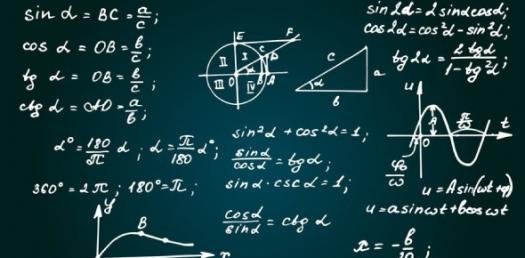# Maths Easy Basic Facts

Approved & Edited by ProProfs Editorial Team
The editorial team at ProProfs Quizzes consists of a select group of subject experts, trivia writers, and quiz masters who have authored over 10,000 quizzes taken by more than 100 million users. This team includes our in-house seasoned quiz moderators and subject matter experts. Our editorial experts, spread across the world, are rigorously trained using our comprehensive guidelines to ensure that you receive the highest quality quizzes.
| By Mr.Sahni
M
Mr.Sahni
Community Contributor
Quizzes Created: 1 | Total Attempts: 57,021
Questions: 8 | Attempts: 57,021SettingsThe quiz below is perfect for the ages of 10-13. It tests out how well you understand some basic math facts. Do you think you can tackle it with ease? If yes, give it a try and share how high your score is in the comment section. All the best!

• 1.

### 8 Times 4

• A.

8

• B.

4

• C.

32

• D.

24

• E.

36

C. 32
Explanation
The given calculation is 8 times 4. Multiplying 8 by 4 gives the result of 32.

Rate this question:

• 2.

### 60 Divided By 10

• A.

10

• B.

60

• C.

7

• D.

6

D. 6
Explanation
The correct answer is 6 because when you divide 60 by 10, you are essentially asking how many times 10 can go into 60. Since 10 can go into 60 six times (10 x 6 = 60), the answer is 6.

Rate this question:

• 3.

### 12 Divided By 3

• A.

5

• B.

4

• C.

3

• D.

2

• E.

8

B. 4
Explanation
The correct answer is 4 because when you divide 12 by 3, you get 4 as the quotient.

Rate this question:

• 4.

### 7 Plus 14

• A.

14

• B.

9

• C.

21

• D.

28

C. 21
Explanation
The given sequence is formed by adding 7 to the previous number. Starting with 14, adding 7 gives 21.

Rate this question:

• 5.

### 18 plus 64

• A.

80

• B.

82

• C.

84

• D.

86

B. 82
Explanation
The given question is asking for the sum of 18 and 64. Adding these two numbers together gives us a total of 82.

Rate this question:

• 6.

### 17-9

• A.

7

• B.

8

• C.

9

• D.

5

B. 8
Explanation
The given expression is 17-9. When we subtract 9 from 17, we get the result 8. Therefore, the correct answer is 8.

Rate this question:

• 7.

### 81 Minus 4

• A.

72

• B.

73

• C.

77

• D.

78

C. 77
Explanation
The correct answer is 77 because when you subtract 4 from 81, you get 77.

Rate this question:

• 8.

### 99 Plus 1 Then Times 4 Then Minus 1

• A.

338

• B.

399

• C.

400

• D.

323

B. 399
Explanation
To solve the given expression, we follow the order of operations (PEMDAS). First, we add 99 and 1, which gives us 100. Then, we multiply 100 by 4, resulting in 400. Finally, we subtract 1 from 400, giving us the final answer of 399.

Rate this question:

Related TopicsBack to top